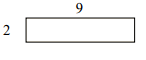# Distinguishing between the area and perimeter of a rectangle

In this lesson we solve real world problems involving the perimeter and area of rectangles. This makes it easy to distinguish between the practical application of perimeter and area calculation of rectangles in real life.

Formula for finding Perimeter of a rectangle

The perimeter of a rectangle of length l and width w is given by

Perimeter P = 2(l + w) units

Formula for finding Area of a rectangle

If a rectangle has a length ‘l’ and a width ‘w’, then the area of the rectangle is given by

Area A = l × w square units

Find the perimeter of the following rectangle.### Solution

Step 1:

Perimeter of a rectangle = 2(l + w); l = length = 6; w = width = 5

Step 2:

Perimeter of given rectangle = 2(6 + 5) = 22 units

Find the area of the following rectangle.### Solution

Step 1:

Area of a rectangle = l × w; l = length = 9; w = width = 2

Step 2:

Area of given rectangle = 9 × 2 = 18 square units